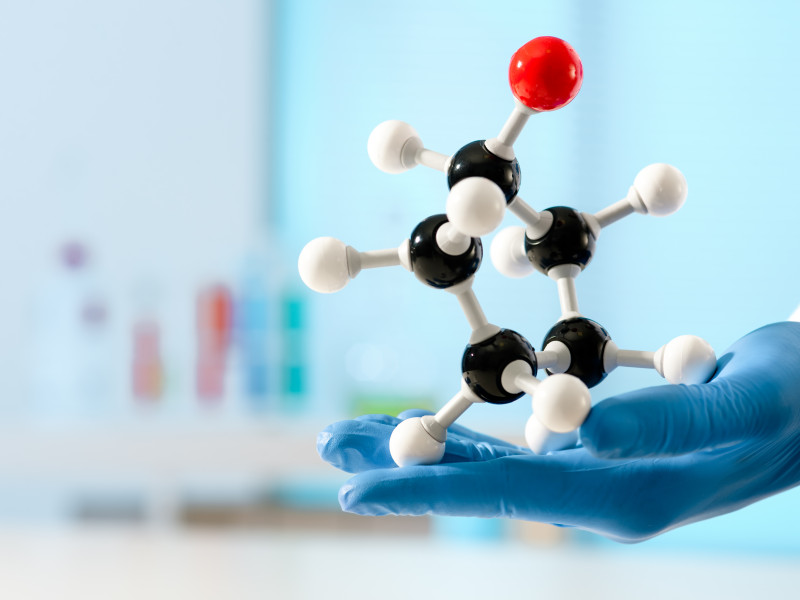# Quantum Computing for Quantum Chemistry (QC4QC)

The simulation of chemical systems is predicted to be one of the most important and killer applications in near-term and fault-tolerant quantum devices (Reiher et al, PNAS, 2017).But there are still many gaps to close before we can witness the power of quantum computers in the chemistry field:

• The hardware needs to improve
• The software needs to be further developed
• The domain knowledge gap needs to be narrowed.

At SURF we support various research institutes and universities of the Netherlands and the chemistry community is currently the largest group of users.

Within this project we aim to help in the integration and interaction of the different fields involved in quantum computing for quantum chemistry, while at the same time contributing to the overall understanding, development, and improvement of current algorithms and their implementations.

## The problem

Quantum chemistry aims to use computers to approximate the electronic wave function of atoms and molecules to extract useful information about real chemical systems. In general, the electronic wave function we are after is that associated with the molecule's ground state. Ground state electron configurations are the foundation for understanding molecular bonding, properties, and structures.

Given a set of atoms, the problem consists of trying to find the electron configuration that minimizes the
energy of a system. Atoms are in general assumed to be static.Quantum computers are two-level quantum
systems (qubits) which can be initialized, sufficiently controlled, and measured, in order to perform a computational task.

Given a set of atoms (here represented by the blue charges), there can be many different electron configurations (represented by the yellow, red and orange cloud). The problem consists of trying to find the configuration that minimizes the energy of a system (represented by the blue cloud). Atoms are in general assumed to be static as they move much slower then electrons.

For quantum chemistry most of the quantum algorithms (a sequence of quantum gates to solve a specific problem) are designed to estimate the ground state energies of molecular systems. This task can be done using:

• Quantum Phase Estimation Algorithm (QPEA)
• Variational Quantum Eigensolver (VQE)

QPEA success relies on all the operations being executed fully coherently on the quantum computer, which is only possible using quantum error correction. This requirement is unattainable on NISQ devices because the operations available on these devices are too error-prone to achieve useful quantum error correction (Y. Cao et al, ChemRev 2019). Therefore in this project we focus on VQE.

## VQE

Variational quantum algorithms utilize a hybrid approach involving a quantum computer and a classical computer working in tandem. Specifically, VQE “measures” the energy of a given parametrized quantum state in the quantum computer but undergoes the parameters optimization process in a classical computer.

In a variational algorithm the parameters of the problem are varied in order to find the values for which the cost function is optimal. Here we are trying to find the shape of the stick for which the energy (cost function) is optimal (minimum). In each cycle we change some parameters (eg. number of bends, size of the bends) and we compute the energy. If the change in the energy is favorable (i.e the energy decreases), we keep the change, otherwise we disregard it. This process is performed iteratively until the energy decrease with variations in the parameters becomes negligible. At this point we assume we have reached the optimal value (minimum). In VQE the energy is computed in the quantum computer but the optimization of the parameters is performed using a classical routine in a classical computer. The measured energy by the quantum computer is used by the classical routine to decide in which direction to change the parameters and the optimized parameters are used to modify the circuit by modifying the parametrized gates (eg. the angle of rotation gates).

An initial guess state dependent on a set of parameters is initialized in the quantum computer and by applying the
quantum algorithm the quantum computer determines its energy. The result of this measurement is sent back to the
classical computer which in turn tunes the parameters of the initial guess based on a classical optimization algorithm to
improve the quality of the state.

## Project goals

1. Provide universities with a development and execution environment for quantum computing software
2. Support the development of interfaces between OpenFermion and quantum software used by our community
3. Research and improvement regarding the classical optimization algorithms for VQE
4. Optimize design of VQE
5. Create and share workflows for quantum chemistry using quantum computing algorithms